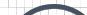Informative Information for the Uninformed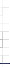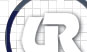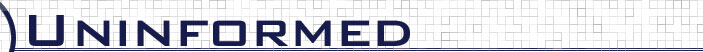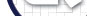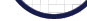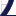Vol 1» 2005.May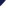# Timing Graph

Sometimes it really helps to see data graphically instead of just a bunch of hex and 1's and 0's, so my friend pr0le threw together this perl script that creates an image with a time diagram of the lines. By analyzing this it made it easier to see how they were performing reads and writes to the card.

```#!/usr/bin/perl
use GD;

my \$logfile = shift || die "usage: \$0 <logfile>\n";

open( F, "<\$logfile" );
my @lines = <F>;
close( F );

my \$len = 3;

my \$im_len = scalar( @lines );
my \$w = \$im_len * \$len;
my \$h = 100;

my \$im = new GD::Image( \$w, \$h );
my \$white = \$im->colorAllocate( 255, 255, 255 );
my \$black = \$im->colorAllocate(   0,   0,   0 );

\$im->fill( 0, 0, \$white );

my \$i   = 1;
my \$init = 0;
my (\$bit1,\$bit2,\$rst,\$clk);
my (\$lbit1,\$lbit2,\$lrst,\$lclk) = (undef,undef,undef,undef);
my (\$x1, \$y1, \$x2, \$y2);
foreach my \$line ( @lines ) {
(\$bit1,\$bit2,\$rst,\$clk) = (\$line =~ m/^(\d)(\d)(\d)(\d)/);
if( \$init ) {
&print_bit( \$lbit1, \$bit1, 10 );
&print_bit( \$lbit2, \$bit2, 30 );
&print_bit( \$lrst,  \$rst, 50 );
&print_bit( \$lclk,  \$clk, 70 );
}
(\$lbit1,\$lbit2,\$lrst,\$lclk) = (\$bit1,\$bit2,\$rst,\$clk);
\$init = 1;
\$i++;
}

open( F, ">\$logfile.jpg" );
binmode F;
print F \$im->jpeg;
close( F );

exit;

sub print_bit {
my (\$old, \$new, \$ybase) = @_;

if( \$new != \$old ) {
if( \$new ) {
\$im->line( \$i*\$len, \$ybase+10, \$i*\$len, \$ybase+20, \$black );
\$im->line( \$i*\$len, \$ybase+20, \$i*\$len+\$len, \$ybase+20, \$black );
} else {
\$im->line( \$i*\$len, \$ybase+20, \$i*\$len, \$ybase+10, \$black );
\$im->line( \$i*\$len, \$ybase+10, \$i*\$len+\$len, \$ybase+10, \$black );
}
} else {
if( \$new ) {
\$im->line( \$i*\$len, \$ybase+20, \$i*\$len+\$len, \$ybase+20, \$black );
} else {
\$im->line( \$i*\$len, \$ybase+10, \$i*\$len+\$len, \$ybase+10, \$black );
}
}

return;
}
```